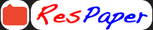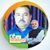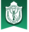Trending ▼   ResFinder# CBSE Class 10 Sample / Model Paper 2021 : Mathematics

9 pages, 36 questions, 0 questions with responses, 0 total responses,00Santosh Jaiswal Delhi Public School (DPS), Indore
+Fave Message
 Home > vikas1239 >Formatting page ...

PRE-BOARD EXAMINATION 2020-21 SUBJECT MATHEMATICS - STANDARD Class: X (CBSE) Total Marks: 80 Date: . Time: 3 hours _______________________________________________________________ General Instructions: 1. This question paper contains two parts A and B. 2. Both part A and part B have internal choices. Part-A: 1. It consists two sections-I and II. 2. Section I has 16 questions of 1 mark each. Internal choices are provided in 5 questions. 3. Section II has 4 case study-based questions. Each case study has 5 case-based subparts. An examinee is to attempt any 4 out 5 sub-parts. Part-B: 1. Question No 21 to 26 are Very Short Answer Type questions of 2 mark each. 2. Question No 27 to 33 are Short Answer Type questions of 3 marks each. 3. Question No 34 to 36 are Long Answer Type questions of 5 marks each. 4. Internal choice is provided in 2 questions of 2 marks, 2 questions of 3 marks and 1 question of 5 marks. ----------------------------------------------------------------------------------------------------------------------- Part- A Section I Section I has 16 Questions of 1 Mark each. Internal choice is Provided in 5 questions 1. Given that LCM (91, 26) = 182 then, find HCF (91, 26). OR If = 32 , where and are positive integers, then find the value of 2. If one zero of the polynomial ( ) = 5 2 + 13 is reciprocal of the other, then find the value of . 3. If 31 + 43 = 117 and 43 + 31 = 105 then, the find the value of + . 4. For what value of k will the following system of equations have unique solution: 2 + = 1 and 3 5 = 7 Page 1 of 9Formatting page ...Formatting page ...Formatting page ...Formatting page ...Formatting page ...Formatting page ...Formatting page ...Formatting page ...